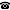# 2017 seminar talk: Slicewise definability in first-order logic with bounded quantifier rank

Talk held by Yijia Chen (Fudan University, Shanghai, People's Republic of China) at the KGRC seminar on 2017-08-17.

### Abstract

For every $q\in \mathbb{N}$ let $\text{FO}_q$ denote the class of sentences of first-order logic $\text{FO}$ of quantifier rank at most $q$. If a graph property can be defined in $\text{FO}_q$, then it can be decided in time $O(n^q)$. Thus, minimizing $q$ has favorable algorithmic consequences. Many graph properties amount to the existence of a certain set of vertices of size $k$. Usually this can only be expressed by a sentence of quantifier rank at least $k$. We use the color-coding method to demonstrate that some (hyper)graph problems can be defined in $\text{FO}_q$, where $q$ is independent of $k$.

It is crucial for our results that the $\text{FO}$-sentences have access to built-in addition and multiplication. It is known that then $\text{FO}$ corresponds to the circuit complexity class uniform $\text{AC}^0$. We explore the connection between the quantifier rank of $\text{FO}$-sentences and the depth of $\text{AC}^0$-circuits, and prove that $\text{FO}_q \subsetneq \text{FO}_{q+1}$ for structures with built-in addition and multiplication.

Kurt Gödel Research Center for Mathematical Logic. Währinger Straße 25, 1090 Wien, Austria.+43-1-4277-50501. Last updated: 2010-12-16, 04:37.